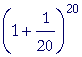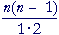An Approach

to

C A L C U L U S

15

# EVALUATINGe

IN THE previous lesson, we saw the following definition of e:On changing the variable from x to 1n , we have:
 e =By letting n take on larger and larger values inwe can come

closer and closer to a decimal value for e.=  2.25=  2.489=  2.594=  2.6534=  2.705=  2.7169

2.7169 is an approximate value for e.

As a more efficient approach, we can derive a sequence that converges to e more rapidly.

 Tolet us apply the binomial theorem:On putting a = 1 and b = 1n , we getNow, e is the limit of that sum as n becomes infinite.  When that happens, each fraction that depends on n approaches 1 because 1 is the quotient of the leading coefficients.  (Lesson 4.)

Therefore, on taking the limit of that sum as n becomes infinite:Notice:  Each term can be derived from the previous term.  The second term follows from the first by dividing it by 1.  The next term follows by dividing by 2.  The next term, by dividing by 3.  The next, by 4.  And so on. e is the limit of the sequence of partial sums.  Here is the sum of the first 10 terms expressed as decimals:

 11st term 1.000000 12nd term (dividing by 1) 1.000000 13rd term (dividing by 2) 0.500000 14th term (dividing by 3) 0.166667 15th term (dividing by 4) 0.041667 16th term (dividing by 5) 0.008333 17th term (dividing by 6) 0.001389 18th term (dividing by 7) 0.000198 19th term (dividing by 8) 0.000025 10th term (dividing by 9) 0.000003 Sum 2.718282

And so after only 10 terms, we obtain a value of e accurate to 6 decimal digits.  That is an example of a rapidly converging series.

e however, like π, is an irrational number.

Problem.   In this term of the binomial theorem,an − 2b2

 show that, on putting a = 1 and b = 1n ,  the term becomesDo the problem yourself first!Please make a donation to keep TheMathPage online.
Even \$1 will help.